# Debug Complexity: How Assertions Affect Debugging Time

Debug Complexity: How Assertions Affect Debugging Time

By Sergey Ignatchenko, Dmytro Ivanchykhin

Debugging any program can be time consuming. Sergey Ignatchenko and Dmytro Ivanchykhin extend their mathematical model to consider the effect of assertions.

Disclaimer: as usual, the opinions within this article are those of ‘No Bugs’ Bunny, and do not necessarily coincide with the opinions of the translators and editors. Please also keep in mind that translation difficulties from Lapine (like those described in [ Loganberry04 ]) might have prevented an exact translation. In addition, the translator and Overload expressly disclaim all responsibility from any action or inaction resulting from reading this article.

In ‘A Model for Debug Complexity’ [ Ignatchenko13 ], we started to build a mathematical model for estimating debugging efforts, and made some sanity checks of our model, in particular on relations between coupling and debug complexity. In this article, we have extended that model to see the effect of the assertions on debugging time. It should be noted that, as previously, the model should be considered to be very approximate, with several assumptions made about the nature of the code and debugging process (though we’re doing our best to outline these assumptions explicitly). Nonetheless, the relations observed within the results obtained look quite reasonable and interesting, which makes us hope that the model we’re working with represents a reasonable approximation of the real world.

## Assumptions

1. In ‘A Model for Debug Complexity’ [II2013], we considered purely linear code. However, it seems that in the context of debugging the same analysis applies to arbitrary code, as long as the execution path is fixed (which is usually the case for deterministic, repeatable debugging); in this case the execution path can be interpreted as linear code for the purposes of analysis. In this article, we’ll use the term ‘linear code’, implying that it is also applicable to any execution path.
2. The linear code consists of (or the equivalent execution path goes through) N lines.
3. In a naive debugging model, the developer goes through the code line by line, and verifies that all the variables are correct. T singlecheck denotes the time to check a single variable.
4. In the earlier article, we mentioned that in many cases it is possible to use a bisection-like optimization to reduce debugging time very significantly. However, such an optimization requires well-defined interfaces to be able to check the whole state of the program easily, and in such cases individual test cases can be easily built to debug an appropriate program part. For the purposes of this article, we will only consider a chunk of code which cannot easily be split into well-defined portions (in other words, a ‘monolithic’ chunk of code), and will not analyze it using bisection optimization.
5. Previously, it was mentioned that not all variables need to be analyzed due to coupling. For the purposes of this article, we’ll use the term ‘variables to be analyzed’; also we expect that for our analysis of chunks of code which cannot be easily split (see item 4 above), the chances of tight coupling are rather high, so the difference between ‘variables’, ‘variables to be analyzed’, and ‘coupled variables’ is not expected to be significant enough to substantially affect the relations observed within our findings.
6. We assume that the number of variables to be changed grows from the beginning to the end of the code; to simplify modeling we also usually assume that this growth is linear.
7. In the earlier article, an obvious optimization – that after the bug is found, the process of going through the code line by line can be stopped – wasn’t taken into account. However, we feel that it doesn’t substantially change relations observed within our findings, and as taking it into account will complicate the mathematics significantly, we’ll leave such analysis for the future.
8. Our analysis is language-independent. That is, all language-specific effects such as ‘in C/C++ you can easily write an assert such as ``` assert(a=b) ``` which will cause bugs’, are out of scope. Also, we’ll use the term ``` ASSERT ``` for assertions in any programming language.
Notation
 N The total number of lines in a ‘monolithic’ code chunk x The current line v(x) The number of variables to consider at line x ; v(x) ~ k*x for some k (see Assumption #6 above) w(x) The amount of work spent on a single line to analyze variables; as discussed in ‘A Model for Debug Complexity’ [ Ignatchenko13 ]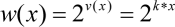W(N) The total amount of work for debugging a single bug in a code of length N ; as it was shown in ‘A Model for Debug Complexity’: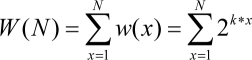This sum may be estimated converting to integrals: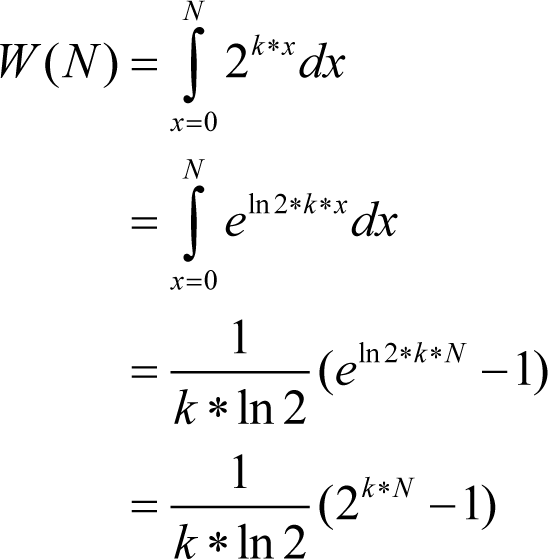which is, if N is large enough is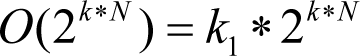with some coefficient k 1 . That is, it grows exponentially with the length of code (NB: we do not consider bisection-like optimization, see Assumption #4 above).

## Introducing ASSERTs

Now assume that there is an ``` ASSERT ``` that catches the bug, defining ‘an ``` ASSERT ``` that catches the bug X ’ as ‘an ``` ASSERT ``` which fails if bug X is present’.

If the ``` ASSERT ``` A is at line x A , then it remains to debug only the first x A lines, and the amount of work required will be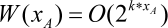It should be pointed out that the ratio of the amount of work without this ``` ASSERT ``` A , compared to that with it, will be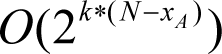This suggests, in particular, that an ``` ASSERT ``` in the middle of code can save far more than 50% of the work.

For instance, in a one-thousand line code chunk with 10 variables to be analyzed at the end (that is, N =1000, and k =0.01) the total amount of work without asserts may be of the order ofAnd with the ``` ASSERT ``` in the middle of the code this value will becomewhich is 33 times less!

In practice, an ``` ASSERT ``` may catch a bug with some probability: one may assume that checking a certain condition in the ``` ASSERT ``` will catch the bug, but indeed, may not. Let’s say that an ``` ASSERT ``` has a probability p A of catching a bug. Then, the expectation of the amount of work may be estimated as a sum of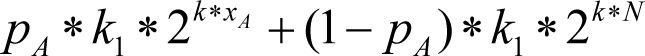where the first term is for the case when the ``` ASSERT ``` is successful, and the second term is for the opposite case.

## Quality of ASSERTs

Clearly, the greater the probability of an ``` ASSERT ``` catching the bug, the less debugging work has to be done. But is it true that an ``` ASSERT ``` with probability of catching a bug of, say, 0.3 is only twice as bad than that with probability 0.6? If two ``` ASSERT ``` s are independent and have a probability of catching a bug of 0.3, then the probability that the bug won’t be caught by either of them is (1-0.3) 2 = 0.49. Let’s use the above example, and assume that all ``` ASSERT ``` s sit in the middle of the code. Substituting, we may get for the ``` ASSERT ``` with probability 0.6: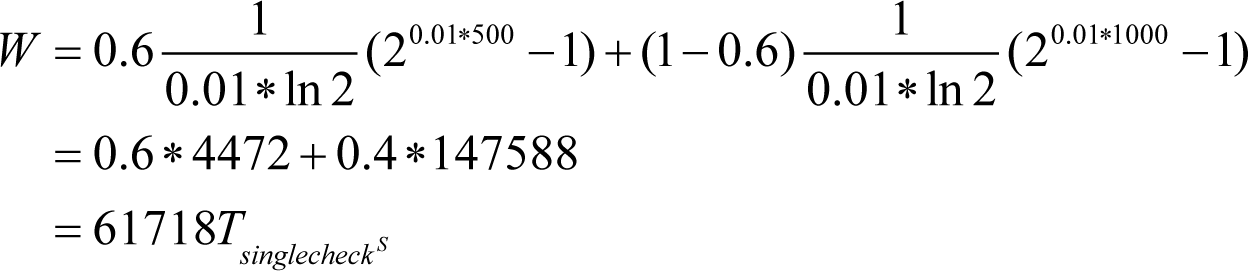And for two ``` ASSERT ``` s with probability 0.3 each:Let’s define an ‘assert which has a high probability of catching probable bugs’ as a ‘high-quality assert’. Unfortunately, there seems to be no simple recipe on ‘how to write high-quality asserts’, though one consideration may potentially help: if an assert aims to catch one of the most common bugs it has quite a good chance of being a ‘high-quality assert’. In particular, ‘No Bugs’ has observed that, when coding in C/C++, simple assertions, say of an array index being within the array boundaries, are extremely rewarding in terms of helping to reduce debugging times – simply because it is very easy to go beyond allocated memory, and it is very difficult to find the exact place where this has happened. Another potential suggestion is related to using asserts as a way of (enforced at runtime) documenting code [Wikipedia]; such ‘code documenting’ asserts (in the experience of ‘No Bugs’) tend to catch more subtle logical bugs.

## Multiple bugs

In general, ``` ASSERT ``` A may catch more than a single bug, and we may talk about the probability p A B of the ``` ASSERT ``` A catching a specific bug B residing in the code. Thus, if there are n bugs, then ``` ASSERT ``` A may have probabilities p A Bi of catching bug Bi for each i from 1 to n . With this assumption, for instance, the probability that ``` ASSERT ``` A is useless is the product:We may call this product the value ineffectiveness of an ``` ASSERT ``` . It is clear to see that if, with time, some bugs are caught and therefore the number of bugs decreases, the value ineffectiveness of ``` ASSERT ``` s tend to increase. Let’s denote it by IL . The complimentary probability, 1- IL , gives a chance that the ``` ASSERT ``` catches at least one bug.

## Multiple bugs – multiple ASSERTs

In a real program, there is often (alas!) more than a single bug, and it is (luckily!) possible to place more than a single ``` ASSERT ``` . Then the amount of work to catch a single bug in a code with n bugs and m ``` ASSERT ``` s placed at lines x i , respectively, may be estimated (assuming for simplicity that ``` ASSERT ``` s are enumerated in the order of lines they are placed at) as: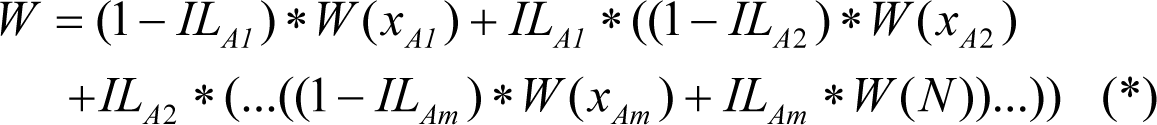For instance, in the above example with three ``` ASSERT ``` s at lines 250, 500, and 750, respectively, and values of ineffectiveness of 0.5 each, to catch a single bug the amount of work will be: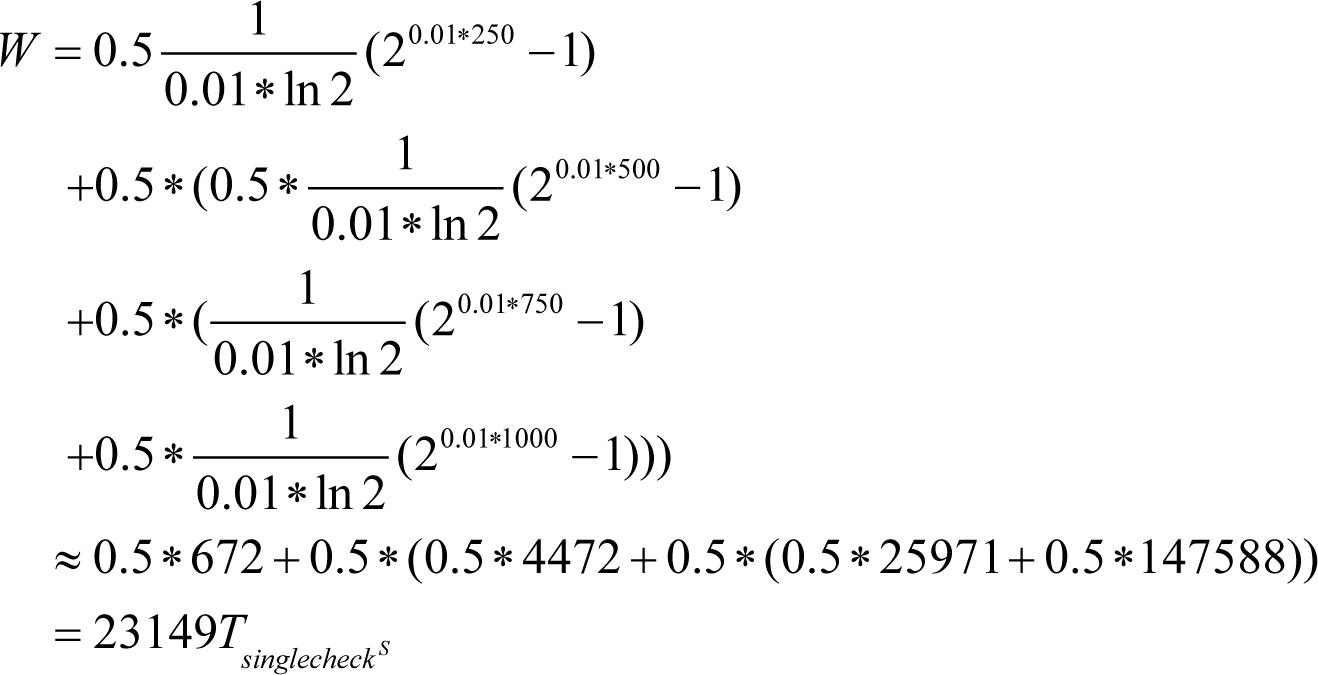which is more than 6 times less than without any ``` ASSERT ``` s.

To illustrate the effect of using asserts from slightly different point of view, for simplicity we may make another assumption that for any ``` ASSERT ``` the probability p of catching any specific bug is the same: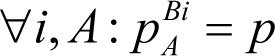Then in the above notation, the value of ineffectiveness IL may be written as a function of number of remaining k bugs:Then, using (*) above, we may calculate the work for finding a bug when only k bugs remain: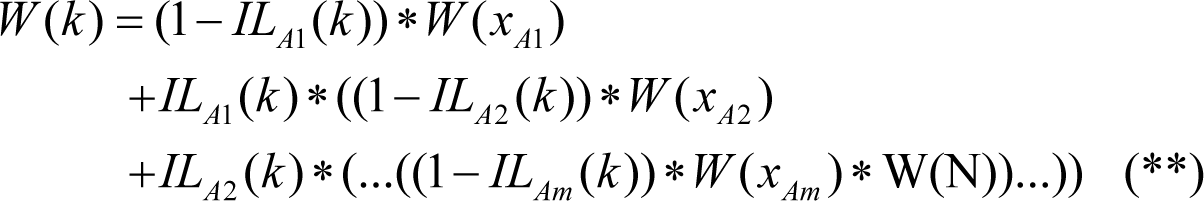Adding up the amounts of work W ( k ) for each k from n to 1 will give us a total expected amount of work to debug all n bugs:To get some taste of what these formulae mean, we have calculated a few samples based on the example that we considered above: a chunk of 1000 lines of ‘monolithic’ code, a linear increase of the number of variables to be analyzed along the code from 1 to 10, 5 bugs, and certain number of ``` ASSERT ``` s with a bit more realistic probability of catching a bug of 0.02; the resulting graph of ‘cumulative amount of work as debug progresses through finding bugs’ is shown on Figure 1.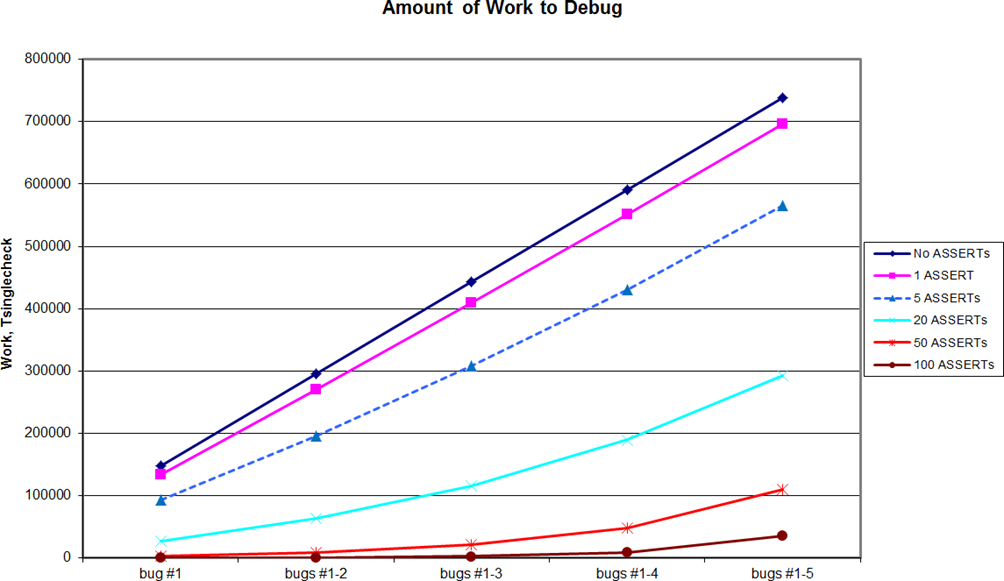Figure 1

In particular, this graph illustrates that, as we have mentioned above, the ``` ASSERT ``` effectiveness tends to ‘degrade’ as debugging goes ahead. This finding is consistent with what we observe in practice, where catching ‘the last bug’ will usually require far more work than the first one. One way that is derived from practical experience, and which follows from the above reasoning, is to add ``` ASSERT ``` s… or to follow a good habit of using them in any place where conditions may be in doubt whilst coding.

Another example of calculation is shown on Figure 2 and illustrates how increasing the number of ``` ASSERT ``` s helps to reduce amount of work necessary to debug the program (note that for presentation purposes, the number of ``` ASSERT ``` s on the graph is near-logarithmic).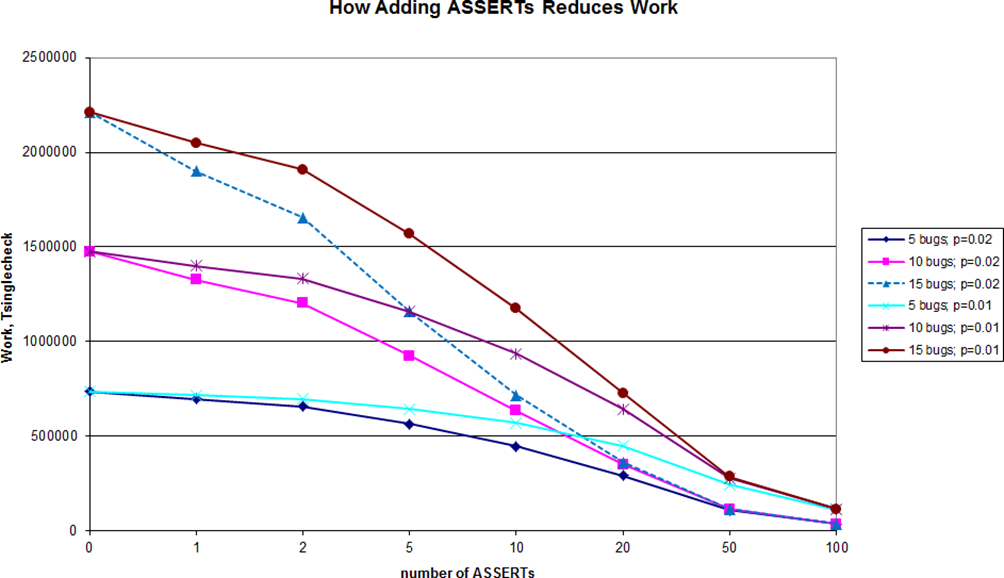Figure 2

Note that while the nature of our analysis is very approximate, the relations observed within our results are expected to be reasonably close to reality; that is, while real-world debugging time can easily differ from the results calculated using our formulae, reduction of the real-world debugging time as number of ``` ASSERT ``` s increases, should be reasonably close to those calculated and shown on the graphs.

## Conclusion

~ Pink Dinosaur from Garfield and Friends

Within the debug complexity model previously introduced [II2013], we have analyzed the impact of asserts on debugging time. Our results seem to be quite consistent with debugging practice:

• ``` ASSERT ``` s can reduce debugging time dramatically (making it several times less)
• debugging-wise, there are ‘high-quality asserts’ and ‘not-so-high-quality asserts’
• purely empirical suggestions for ‘high-quality asserts’ were given in the ‘Quality of ASSERTs’ section
• the time for debugging ‘the last bug’ is significantly higher than the time for debugging the first one.

In addition, it should be noted that the impact of ``` ASSERT ``` s on the program is not limited to a reduction in debugging time. As such effects are well beyond the scope of this paper, we’ll just mention a few of them very briefly. On the negative side: depending on the programming language (and especially for the languages where an ``` ASSERT ``` is a mere function/macro, such as C/C++) it may be possible to write an ``` ASSERT ``` which changes the state of the program (see also Assumption #8 above). On the positive side, ``` ASSERT ``` s can be used to create documentation of the program, where such documentation (unlike, say, comments) cannot become out of date easily.

Overall, ‘No Bugs’ highly recommends the using of ``` ASSERT ``` s, though feels that creating any kind of metrics such as ‘number of ``` ASSERT ``` s per 1000 lines of code’, as a result of Goodhart’s Law [ Goodhart ], will become as useless as ‘number of comments per 1000 lines of code’. As ``` ASSERT(1==1) ``` is as useless as it gets, it is certainly not about sheer numbers, so it is important to use high-quality ``` ASSERT ``` s. This still seems to be an art rather than science, though a few hints for ‘high-quality ``` ASSERT ``` s’ were provided above, and most likely there are many more other such hints in existence.## References

[Loganberry04] David ‘Loganberry’, Frithaes! – an Introduction to Colloquial Lapine!, http://bitsnbobstones.watershipdown.org/lapine/overview.html

[Ignatchenko13] ‘A Model for Debug Complexity’, Sergey Ignatchenko and Dmytro Ivanchykhin, Overload 114, April 2013

[WikiAssertion] http://en.wikipedia.org/wiki/Assertion_(software_development)#Assertions_in_design_by_contract : Assertions can function as a form of documentation: they can describe the state the code expects to find before it runs (its preconditions), and the state the code expects to result in when it is finished running (postconditions); they can also specify invariants of a class.

## Acknowledgement

Cartoons by Sergey Gordeev from Gordeev Animation Graphics, Prague.